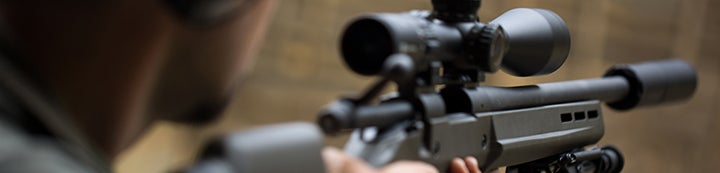1 - 1 of 1 Posts

#### d'Artagnan

·
##### Registered
Joined
·
16 Posts
Discussion Starter · ·
The Federal Cartridge Company has used several lot numbering schemes, which include the encoded manufacture date, on the ammo and primer boxes since at least 1965.
I. 2004-present (2009)
The format is ###L###, where # is a digit and L is a letter. The letter is the year:
H = 2004
Y = 2005
R = 2006
V = 2007
W = 2008
Z = 2009
The digits following the letter are the Julian day plus 400. In the Julian system (a one-up numbering of days 1 thru 365/366) 1 January is day 1, 31 January is day 31, 2 February is day 32, 31 December is day 365, or 366 in a leap year.
The very first number probably is the work shift, numbered 1 thru 3. Occasionally a letter is found in this position, I don't know what it equates to. The next two digits are the production line:
10 = 38 Special
11 = 40 S&W
13 = 44 Magnum
16 =357 Magnum
17 = 38 Special
22 = 9mm Luger
23 = 9mm Luger
24 = 9mm Luger
25 = 45 Auto
26 = 45 Auto
28 = 45 Auto/380 Auto
38 = 45 Auto
39 = Auto
42 = 40 S&W
43 = 9mm Luger
45 = 40 S&W
46 = 45 Auto
47 = 223 Remington
48 = 40 S&W
Examples:
1 42H527 = 1st shift, 40 S&W line 42, 2004, day 127 (6 May)
3 24Y694 = 3rd shift, 45 Auto line 25, 2005, day 294 (21 Oct)
123R756 = 1st shift, 9mm Luger line 23, 2006, day 356 (22 Dec)
246V431 = 2nd shift, 45 Auto line 46, 2007, day 31 (31 Jan)
U43W689 = U=unknown, 9mm Luger line 43, 2008, day 289 (15 Oct)
117Z429 = 1st shift, 38 Special line 17, 2009, day 29 (29 Jan)
II. 2002-2003
###L### format same as 2004-2009, shift and production line numbers the same, Year N probably = 2002, J = 2003; Julian days without adding 400. Examples:
117J064 = 1st shift, 38 Special line 17, 2003, day 64 (5 Mar)
146N213 = 1st shift, 45 Auto line 46, probably 2002, day 213 (1 Aug)
III. 1994-2001
######L### format, except where letter = X where it is #####L###. Letter is year:
H = 1994
Y = 1995
R = 1996
I speculate V = 1997, based on V = 2007 in 2004-2009.
W = possibly 1998
Z = 1999
T = possibly 2000
X = possibly 2001
The three digits following the letter are the Julian day without adding 400. The very first two digits are the production line, same as 2004-2009. The next four digits, or 3 in the case of letter=X, I don't know. Examples:
281623H242 = 380 Auto line 28, 1623=unknown, 1994, day 242 (30 Aug)
095018Y326 = 380 Auto line 09, 5018=unknown, 1995, day 326 (22 Nov)
094992Z105 = 380 Auto line 09, 4992=unknown, 1999, day 105 (15 Apr)
IV. 1992
Similar to 1994-2001 except the year, in the 7th position, is a 2 not a letter and 400 has been added to the Julian day. Examples:
2240352720 = 9mm Luger line 22, 4035=unknown, 1992, day 320 (15 Nov)
3846522530 = 45 Auto line 38, 4652=unknown, 1992, day 130 (9 May)
V. 1984-1989
#L-####, ##L-####, or ##-L#### format. First digit or first two digits probably are the production line; line numbers are probably not the same as 1992-2009. Letter is always A or B, probably for 1st and 2nd shifts. First digit after the hyphen is the last digit of the year: 4 = 1984, 9 = 1989. Last three digits are Julian day plus 400. Examples:
5A-4592 = 5=unknown line, 1st shift, 1984, day 192 (10 Jul)
22A-7682 = 9mm Luger line 22, 1st shift, 1987, day 292 (19 Oct)
22-B9469 = 9mm Luger line 22, 2nd shift, 1989, day 69 (10 Mar)
VI. 1975-1982
Same as 1984-1989 except 400 is not added to Julian day.
VII. 1965
My one example from 1965 appears to have the same numbering scheme as 1984-1989.

1 - 1 of 1 Posts